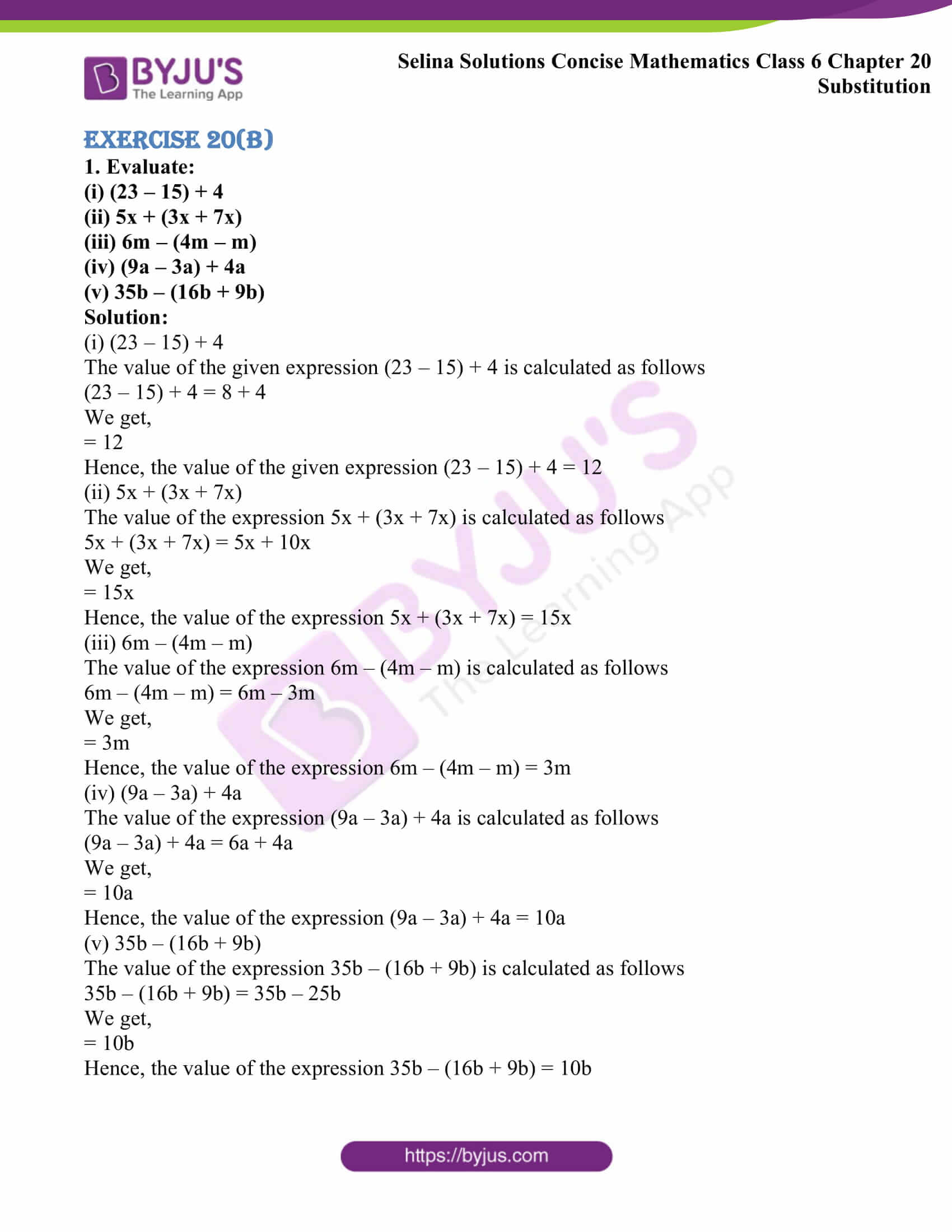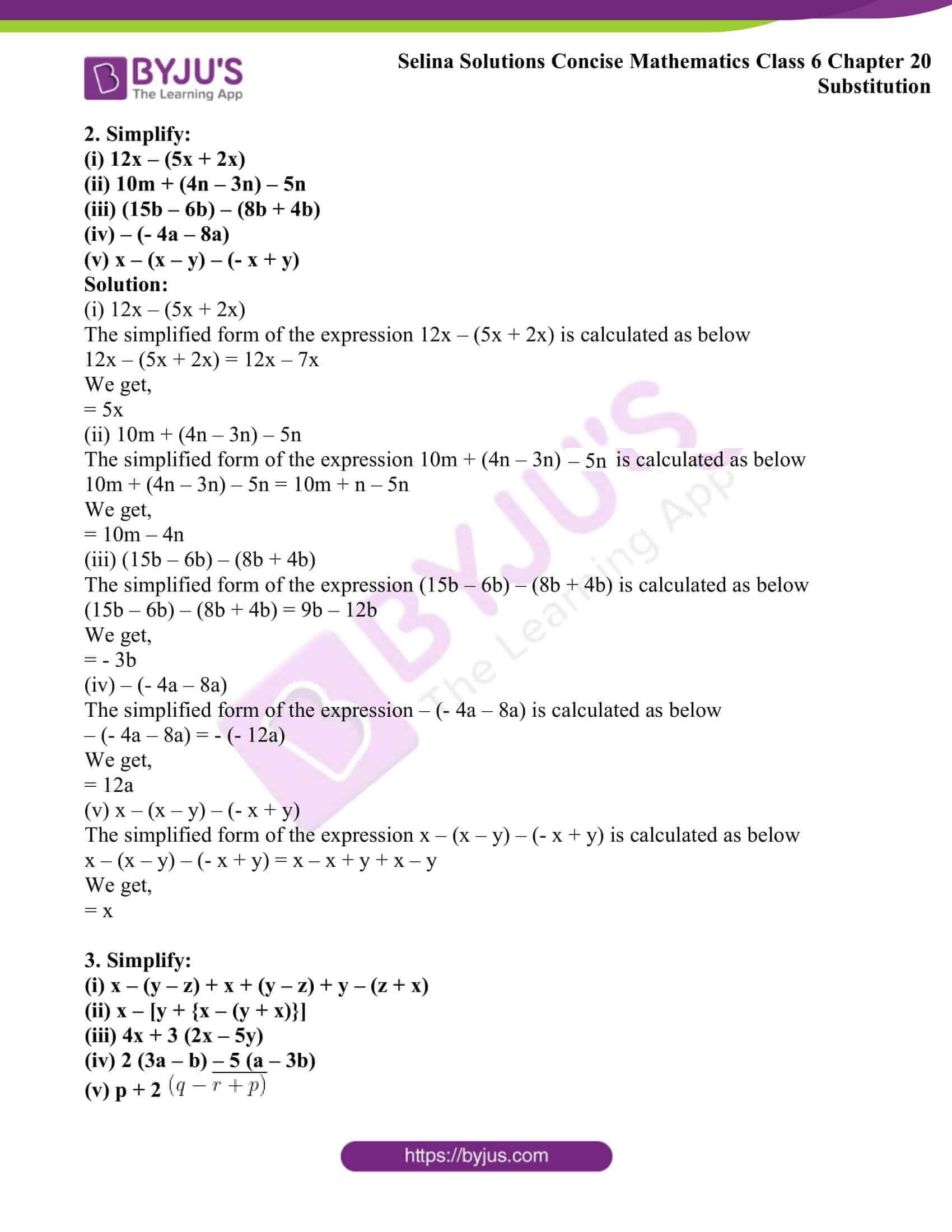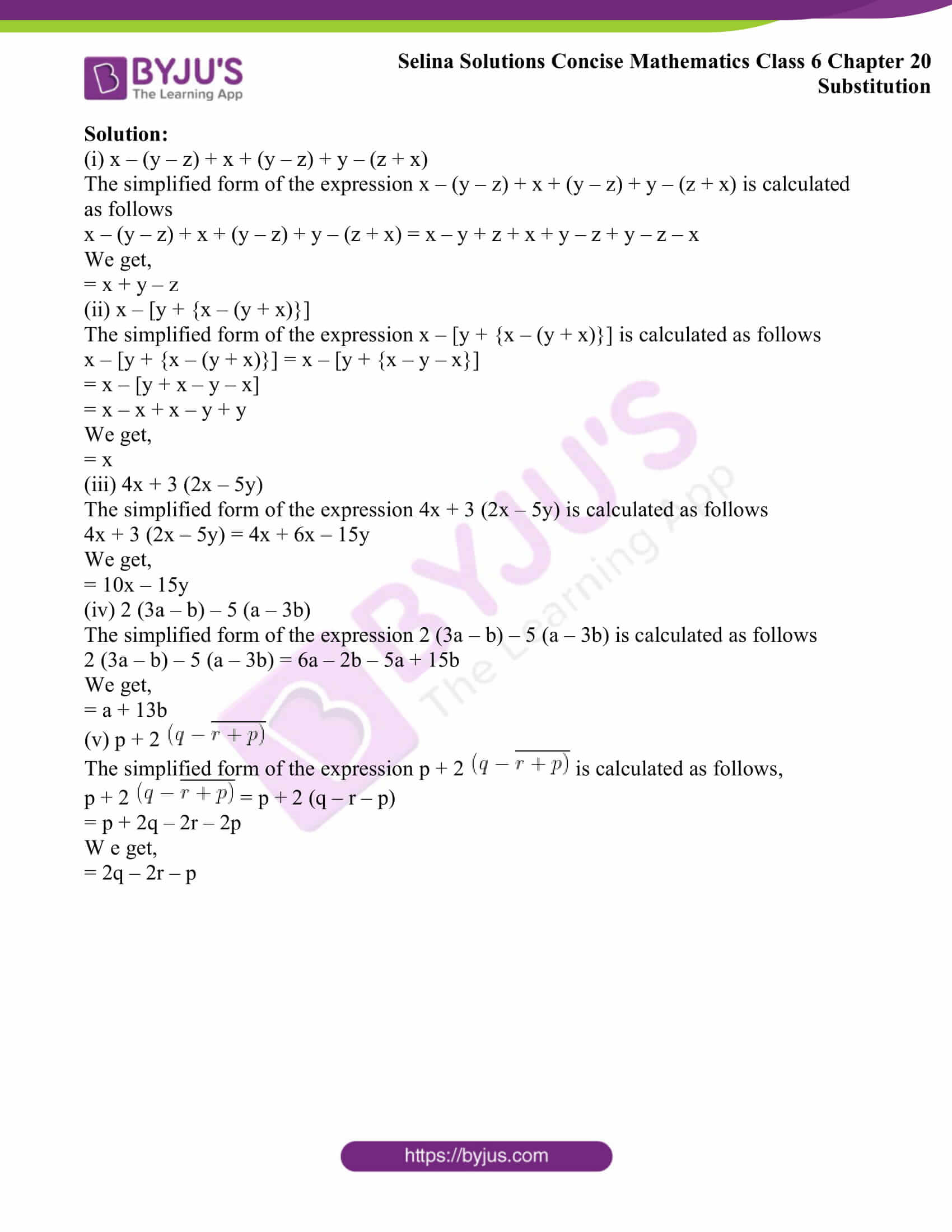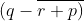# Selina Solutions Concise Mathematics Class 6 Chapter 20 Substitution Exercise 20(B)

Selina Solutions Concise Mathematics Class 6 Chapter 20 Substitution Exercise 20(B) provides students with a clear idea of solving simplification of algebraic expressions with ease, mentioned in this exercise. The solutions are prepared in a simple language, with the aim to make it easy for the students in grasping them. Those who aspire to clear their doubts by themselves can access these solutions both in exercise and chapter-wise format, whenever required. For more detail about the concepts, students can make use of Selina Solutions Concise Mathematics Class 6 Chapter 20 Substitution Exercise 20(B) PDF free, from the links provided here.

## Selina Solutions Concise Mathematics Class 6 Chapter 20 Substitution Exercise 20(B) Download PDF### Access other exercises of Selina Solutions Concise Mathematics Class 6 Chapter 20 Substitution

Exercise 20(A) Solutions

Exercise 20(C) Solutions

### Access Selina Solutions Concise Mathematics Class 6 Chapter 20 Substitution Exercise 20(B)

#### Exercise 20(B)

1. Evaluate:

(i) (23 – 15) + 4

(ii) 5x + (3x + 7x)

(iii) 6m – (4m – m)

(iv) (9a – 3a) + 4a

(v) 35b – (16b + 9b)

Solution:

(i) (23 – 15) + 4

The value of the given expression (23 – 15) + 4 is calculated as follows

(23 – 15) + 4 = 8 + 4

We get,

= 12

Hence, the value of the given expression (23 – 15) + 4 = 12

(ii) 5x + (3x + 7x)

The value of the expression 5x + (3x + 7x) is calculated as follows

5x + (3x + 7x) = 5x + 10x

We get,

= 15x

Hence, the value of the expression 5x + (3x + 7x) = 15x

(iii) 6m – (4m – m)

The value of the expression 6m – (4m – m) is calculated as follows

6m – (4m – m) = 6m – 3m

We get,

= 3m

Hence, the value of the expression 6m – (4m – m) = 3m

(iv) (9a – 3a) + 4a

The value of the expression (9a – 3a) + 4a is calculated as follows

(9a – 3a) + 4a = 6a + 4a

We get,

= 10a

Hence, the value of the expression (9a – 3a) + 4a = 10a

(v) 35b – (16b + 9b)

The value of the expression 35b – (16b + 9b) is calculated as follows

35b – (16b + 9b) = 35b – 25b

We get,

= 10b

Hence, the value of the expression 35b – (16b + 9b) = 10b

2. Simplify:

(i) 12x – (5x + 2x)

(ii) 10m + (4n – 3n) – 5n

(iii) (15b – 6b) – (8b + 4b)

(iv) – (- 4a – 8a)

(v) x – (x – y) – (- x + y)

Solution:

(i) 12x – (5x + 2x)

The simplified form of the expression 12x – (5x + 2x) is calculated as below

12x – (5x + 2x) = 12x – 7x

We get,

= 5x

(ii) 10m + (4n – 3n) – 5n

The simplified form of the expression 10m + (4n – 3n) – 5n is calculated as below

10m + (4n – 3n) – 5n = 10m + n – 5n

We get,

= 10m – 4n

(iii) (15b – 6b) – (8b + 4b)

The simplified form of the expression (15b – 6b) – (8b + 4b) is calculated as below

(15b – 6b) – (8b + 4b) = 9b – 12b

We get,

= – 3b

(iv) – (- 4a – 8a)

The simplified form of the expression – (- 4a – 8a) is calculated as below

– (- 4a – 8a) = – (- 12a)

We get,

= 12a

(v) x – (x – y) – (- x + y)

The simplified form of the expression x – (x – y) – (- x + y) is calculated as below

x – (x – y) – (- x + y) = x – x + y + x – y

We get,

= x

3. Simplify:

(i) x – (y – z) + x + (y – z) + y – (z + x)

(ii) x – [y + {x – (y + x)}]

(iii) 4x + 3 (2x – 5y)

(iv) 2 (3a – b) – 5 (a – 3b)

(v) p + 2Solution:

(i) x – (y – z) + x + (y – z) + y – (z + x)

The simplified form of the expression x – (y – z) + x + (y – z) + y – (z + x) is calculated as follows

x – (y – z) + x + (y – z) + y – (z + x) = x – y + z + x + y – z + y – z – x

We get,

= x + y – z

(ii) x – [y + {x – (y + x)}]

The simplified form of the expression x – [y + {x – (y + x)}] is calculated as follows

x – [y + {x – (y + x)}] = x – [y + {x – y – x}]

= x – [y + x – y – x]

= x – x + x – y + y

We get,

= x

(iii) 4x + 3 (2x – 5y)

The simplified form of the expression 4x + 3 (2x – 5y) is calculated as follows

4x + 3 (2x – 5y) = 4x + 6x – 15y

We get,

= 10x – 15y

(iv) 2 (3a – b) – 5 (a – 3b)

The simplified form of the expression 2 (3a – b) – 5 (a – 3b) is calculated as follows

2 (3a – b) – 5 (a – 3b) = 6a – 2b – 5a + 15b

We get,

= a + 13b

(v) p + 2The simplified form of the expression p + 2is calculated as follows,

p + 2= p + 2 (q – r – p)

= p + 2q – 2r – 2p

W e get,

= 2q – 2r – p